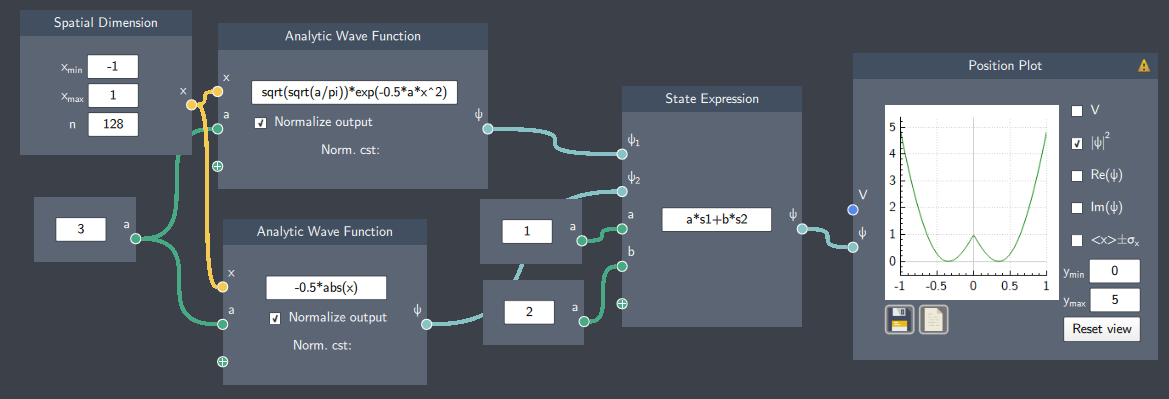# State Expression

## Description

In this node, an expression for a state can be obtained by combining two wave functions with an arithmetic operation.

## Inputs

The node has the following inputs:

• Wave functions ($\psi_{1}$ and $\psi_{2}$) : Two wave functions denoted as "s1"  and "s2" in the input field.
• Scalars ($a$, $b$  etc.) - It is possible to input scalar quantities to parameterize the expression.

## Content

In the content field, an arithmetic operation is defined to combine the two wave functions entered as inputs.

## Output

• Wave Function ($\psi$): The combined state or wave function generated from the expression in the content field.

## Example

An example is shown below where two wave functions (Gaussian and absolute value functions) are added together to plot the resulting wave function.

This is especially helpful if one would like to see an overview of the system they are working with if the desired wave function is a combination of many functions.